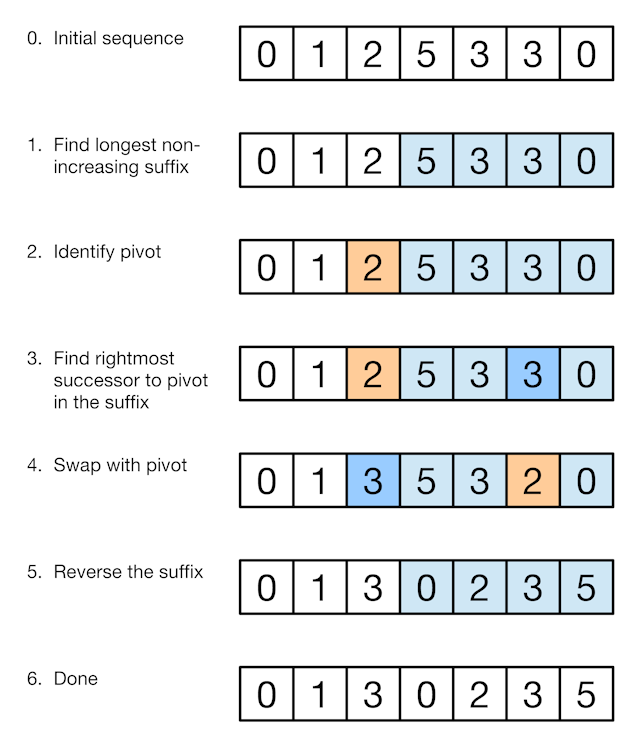# 凌云的博客

## LeetCode 算法题 31. 下一个排列

### 题目

``````1,2,3 → 1,3,2
3,2,1 → 1,2,3
1,1,5 → 1,5,1
``````

### 解法• 找到最大的下标 i 使得 array[i - 1] < array[i]
• 找到最大的下标 j 使得 j > i 并且 array[j] > array[i - 1]
• 交换 array[j] 和 array[i - 1]
• 反转 i 开始的后缀数组
``````class Solution {
public:
void nextPermutation(vector<int>& nums) {
if (nums.empty()) return;

// in reverse order, find the first number which is in increasing trend (we call it violated number here)
int i;
for (i = nums.size() - 2; i >= 0; --i) {
if (nums[i] < nums[i+1]) break;
}

// reverse all the numbers after violated number
reverse(begin(nums)+i+1, end(nums));
// if violated number not found, because we have reversed the whole array, then we are done!
if (i == -1) return;
// else binary search find the first number larger than the violated number
auto itr = upper_bound(begin(nums)+i+1, end(nums), nums[i]);
// swap them, done!
swap(nums[i], *itr);
}
};
``````